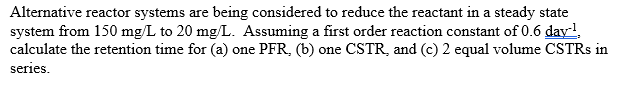# (Solved): Alternative reactor systems are being considered to reduce the reactant in a steady state system f ...Alternative reactor systems are being considered to reduce the reactant in a steady state system from $$150 \mathrm{mg} / \mathrm{L}$$ to $$20 \mathrm{mg} / \mathrm{L}$$. Assuming a first order reaction constant of $$0.6 \mathrm{day}^{-1}$$ : calculate the retention time for (a) one PFR, (b) one CSTR, and (c) 2 equal volume CSTRs in series.

We have an Answer from Expert